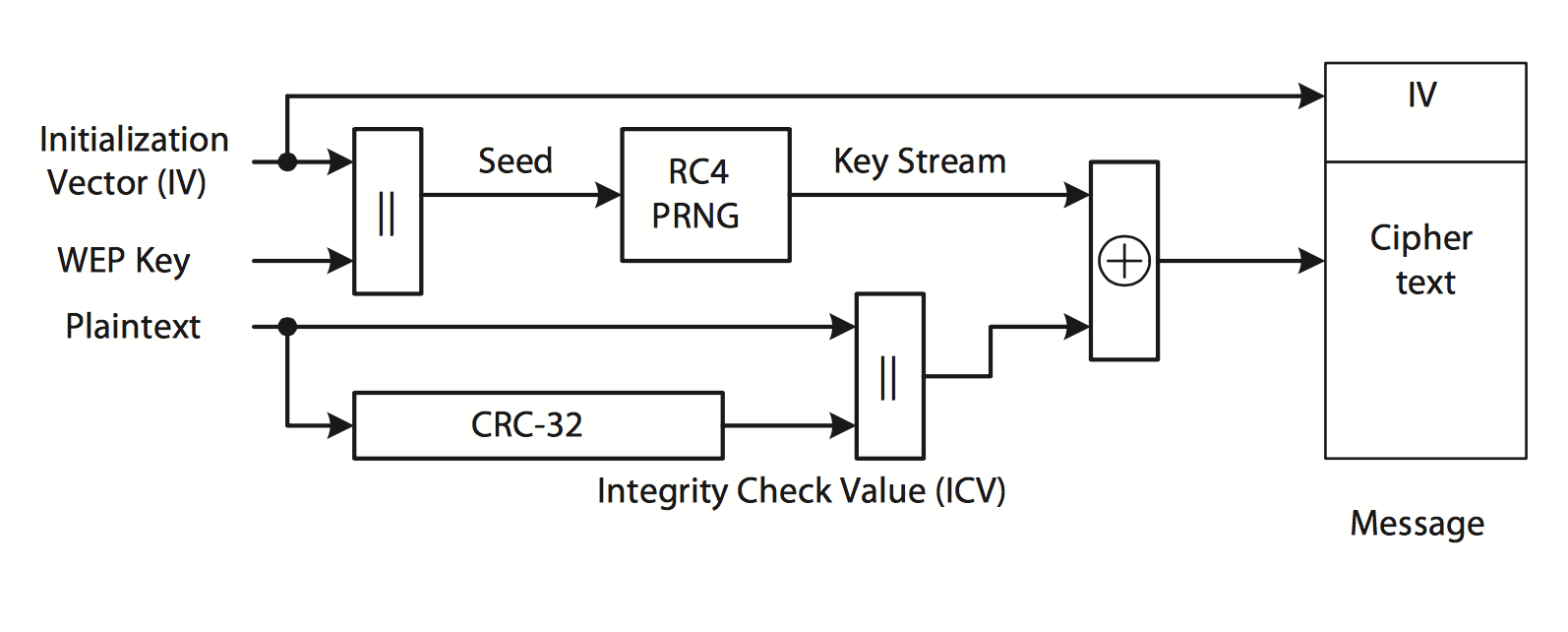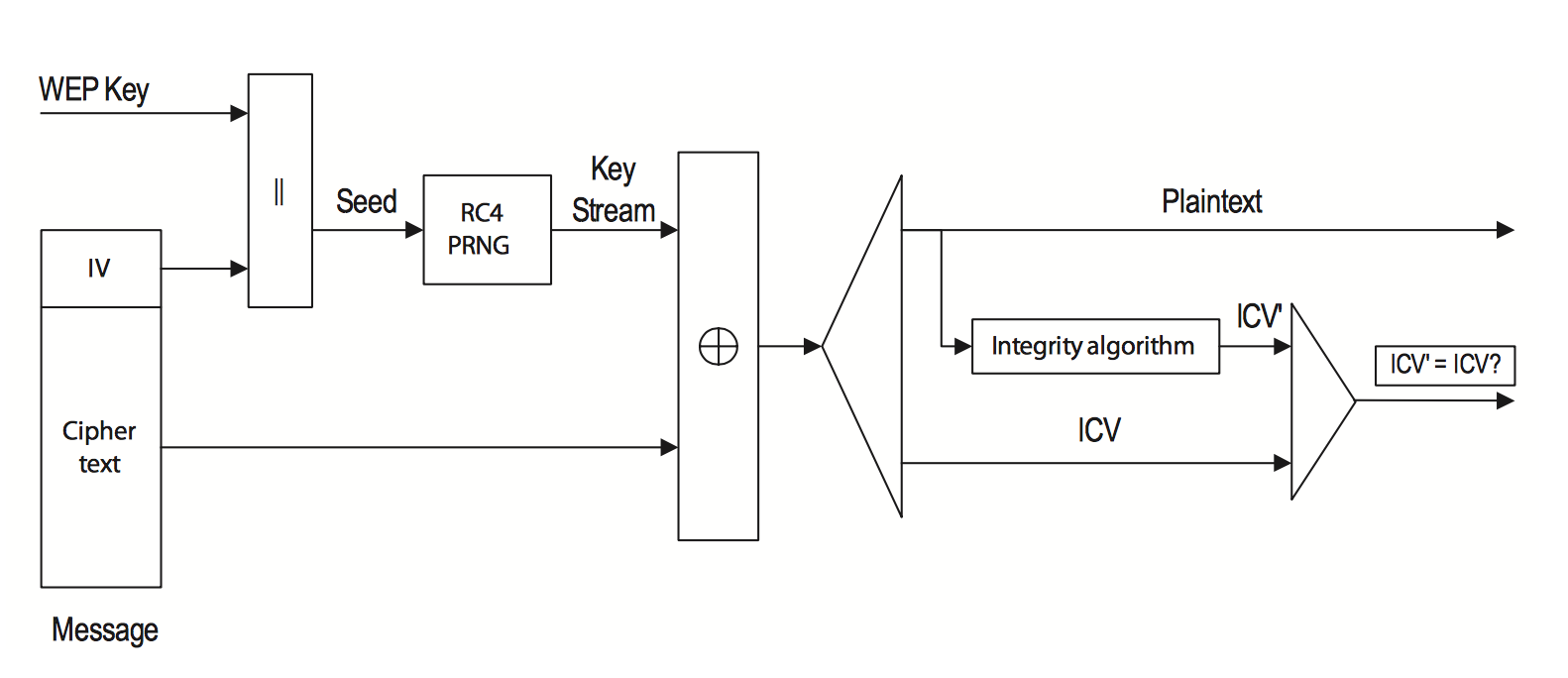# 密码学基础总结-03

## 什么是现代密码学

• 在二进制位上进行操作，这样简单的针对特定语言的频率攻击将无效化，二进制不依赖于特定的人类语言。
• 保护密钥，不保密算法，算法应该公开接受全地球人的检验攻击，如果大家都认为这个算法好，攻击不动才能使用，这也就是柯克霍夫原则（Kerckhoffs’s principle，也称为柯克霍夫假说、公理、或定律），这样才能保证安全性，也可以延伸概念到开源软件这个概念中，开源万岁！
• 同一明文多次加密，每次的密文均不相同，这条我认为也应该算在现代密码学相对于古典密码学的区别中，只有这样才能抵抗选择明文攻击。

## 对称加密

### 加密算法设计的两种基本策略

#### 流密码(序列密码,Stream Cipher)

◼ 将密钥扩展到与密文等长 ◼ 加密算法一般为异或操作 ◼ 其安全性主要在于密钥扩展后的随机性 $M = m 1 m_2 m_3 …, K = k , C = c$ 其中 $c = Enc{k} ( M )$

#### 分组密码(Block Cipher)

◼ 将明文分成等长的分组(比如64位、128位) ◼ 对每个分组采用相同的加密算法进行加密 ◼ 算法一般是交替使用混乱和扩散技术 $M = m 1 m_2 m_3 …., K = k_1 k_2 k_3 …, C = c_1 c_2 c_3 …$ 其中 $c_i = Enc{k_i} ( m_i )$

## 流密码与伪随机数

### 序列加密算法

1. Gen $n→k,k$是一个$[0,2^n]$均匀分布中随机取出的一个整数。
2. Enc $(k, m) → c$,$c\leftarrow G ( k ) \oplus m$
3. Dec $(k, c) → m$; $m \leftarrow G ( k ) \oplus c$

### RC4序列加密算法

##### 伪随机数发生器

i := j := 0
while GeneratingOutput:
i := (i + 1) mod 256
j := (j + S[i]) mod 256
swap values of S[i] and S[j]
SR:= S[(S[i] + S[j]) mod 256]
output SR
endwhile


### WEP加密方案WEP方案加密WEP方案解密

IV就是初始的随机数，||表示简单拼接，CRC-32是循环冗余检验，不在这个地方讨论，Plaintext是明文，$\oplus$ 是异或运算，RC4 PRNG就是上面说的RC4算法。

#### 缺陷

• WEP数据帧中第一个字节消息相同,攻击者可以得到第一个字节的G(k)值。
• IV只有24bits,$2^{12}$个消息就很可能出现碰撞。（生日攻击）
• 利用碰撞,相对容易猜测密文对应的明文。